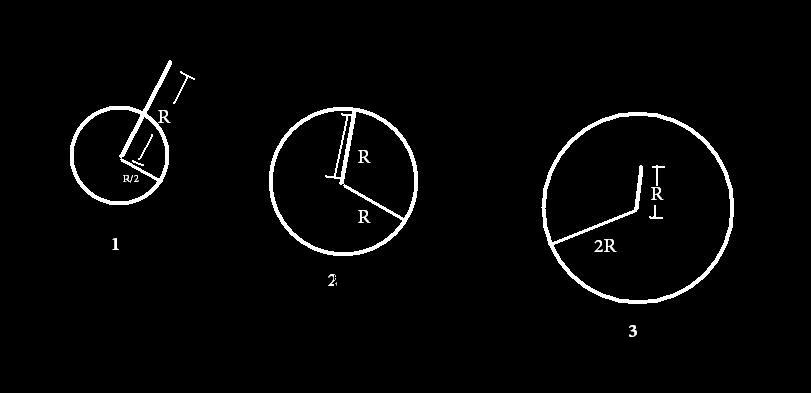# Electrostatics..#1Charges q, 2q, 4q are uniformly distributed in three dielectric solid spheres 1, 2 & 3 at radii R/2, R, & 2R respectively as shown in above figure. If magnitude of the electric field at point P at distance ‘R’ from centre of the spheres 1, 2 & 3 are $E_{1}, E_{2} , E_{3}$ respectively. Then,